Скачать презентацию Relative Valuation Valuing a company relative to another

7d7d1986900198a4bdc44fc1af5c5ba9.ppt

• Количество слайдов: 29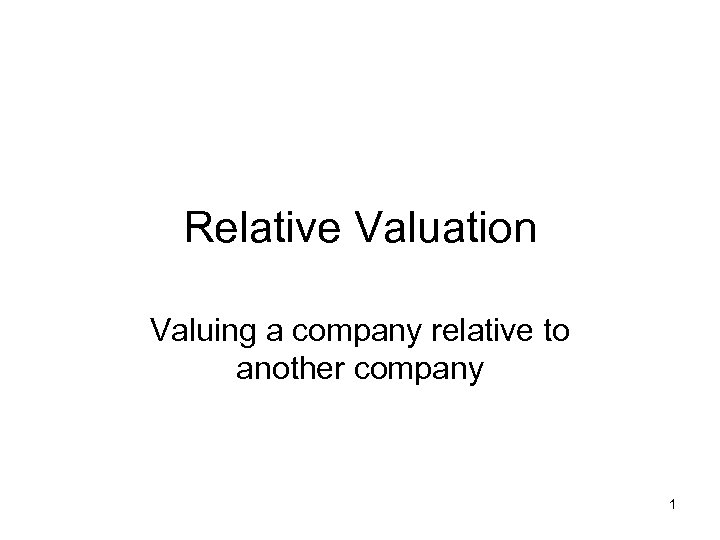Relative Valuation Valuing a company relative to another company 1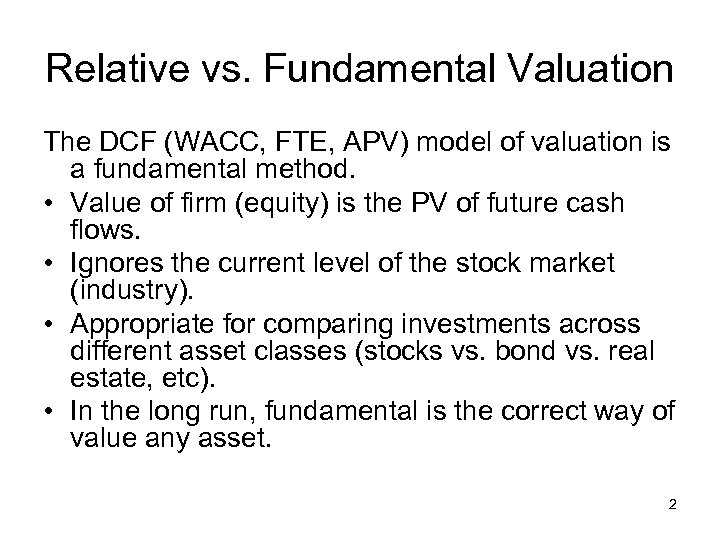Relative vs. Fundamental Valuation The DCF (WACC, FTE, APV) model of valuation is a fundamental method. • Value of firm (equity) is the PV of future cash flows. • Ignores the current level of the stock market (industry). • Appropriate for comparing investments across different asset classes (stocks vs. bond vs. real estate, etc). • In the long run, fundamental is the correct way of value any asset. 2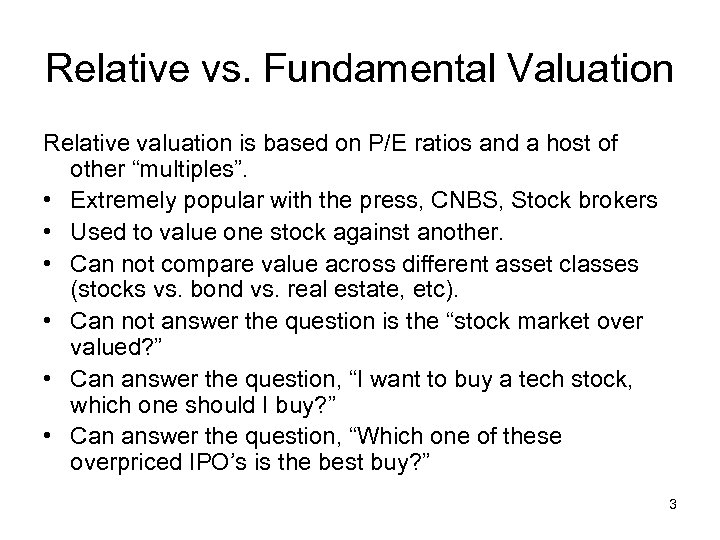Relative vs. Fundamental Valuation Relative valuation is based on P/E ratios and a host of other “multiples”. • Extremely popular with the press, CNBS, Stock brokers • Used to value one stock against another. • Can not compare value across different asset classes (stocks vs. bond vs. real estate, etc). • Can not answer the question is the “stock market over valued? ” • Can answer the question, “I want to buy a tech stock, which one should I buy? ” • Can answer the question, “Which one of these overpriced IPO’s is the best buy? ” 3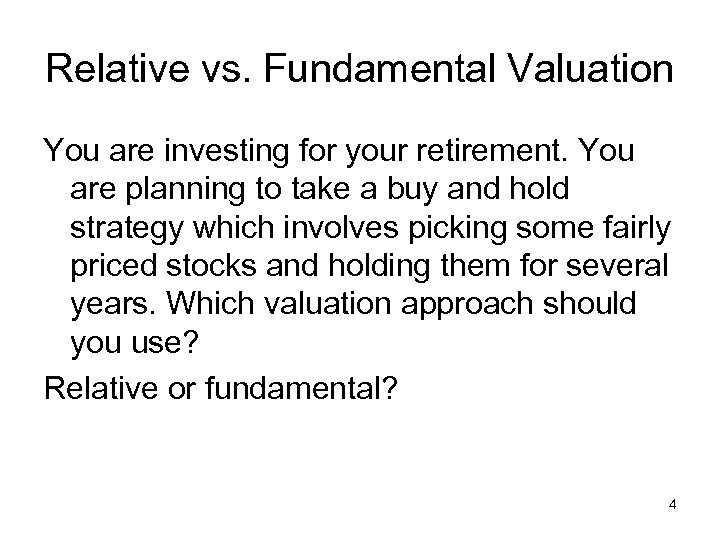Relative vs. Fundamental Valuation You are investing for your retirement. You are planning to take a buy and hold strategy which involves picking some fairly priced stocks and holding them for several years. Which valuation approach should you use? Relative or fundamental? 4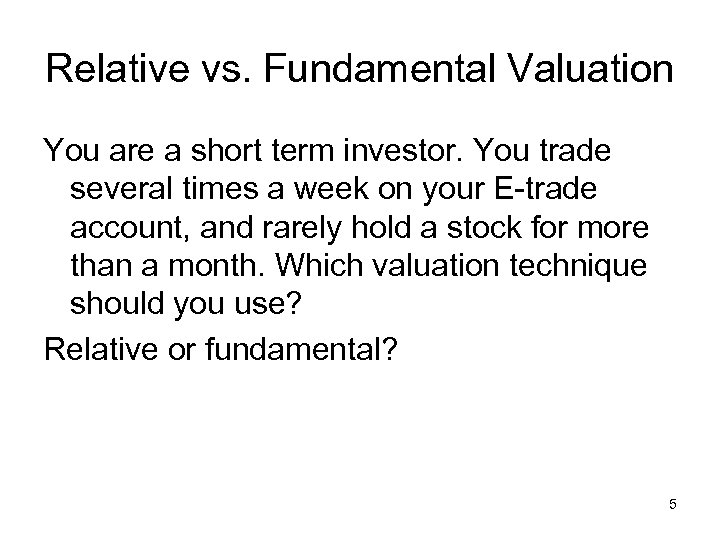Relative vs. Fundamental Valuation You are a short term investor. You trade several times a week on your E-trade account, and rarely hold a stock for more than a month. Which valuation technique should you use? Relative or fundamental? 5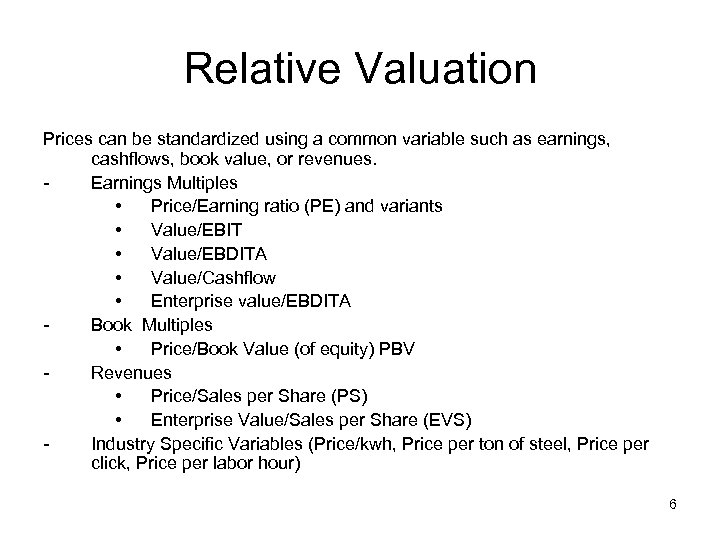Relative Valuation Prices can be standardized using a common variable such as earnings, cashflows, book value, or revenues. Earnings Multiples • Price/Earning ratio (PE) and variants • Value/EBIT • Value/EBDITA • Value/Cashflow • Enterprise value/EBDITA Book Multiples • Price/Book Value (of equity) PBV Revenues • Price/Sales per Share (PS) • Enterprise Value/Sales per Share (EVS) Industry Specific Variables (Price/kwh, Price per ton of steel, Price per click, Price per labor hour) 6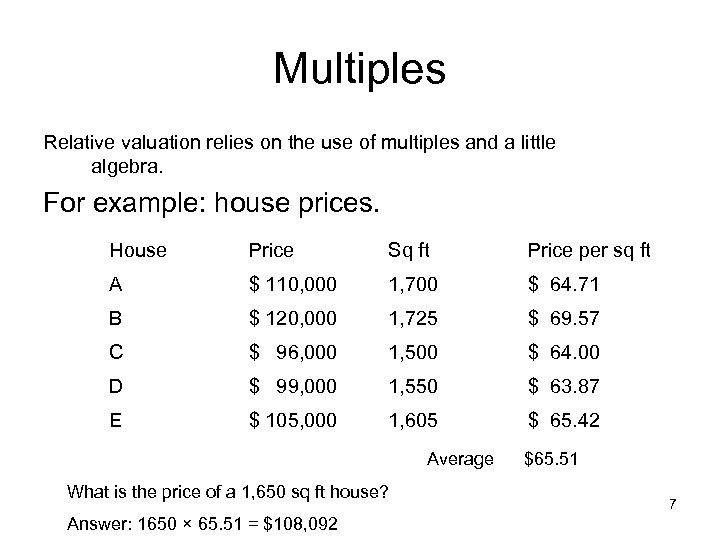Multiples Relative valuation relies on the use of multiples and a little algebra. For example: house prices. House Price Sq ft Price per sq ft A \$ 110, 000 1, 700 \$ 64. 71 B \$ 120, 000 1, 725 \$ 69. 57 C \$ 96, 000 1, 500 \$ 64. 00 D \$ 99, 000 1, 550 \$ 63. 87 E \$ 105, 000 1, 605 \$ 65. 42 Average What is the price of a 1, 650 sq ft house? Answer: 1650 × 65. 51 = \$108, 092 \$65. 51 7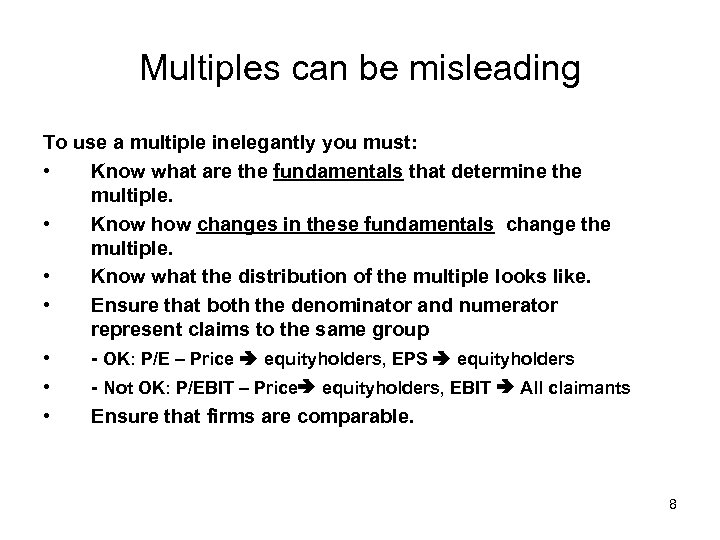Multiples can be misleading To use a multiple inelegantly you must: • Know what are the fundamentals that determine the multiple. • Know how changes in these fundamentals change the multiple. • Know what the distribution of the multiple looks like. • Ensure that both the denominator and numerator represent claims to the same group • - OK: P/E – Price equityholders, EPS equityholders • - Not OK: P/EBIT – Price equityholders, EBIT All claimants • Ensure that firms are comparable. 8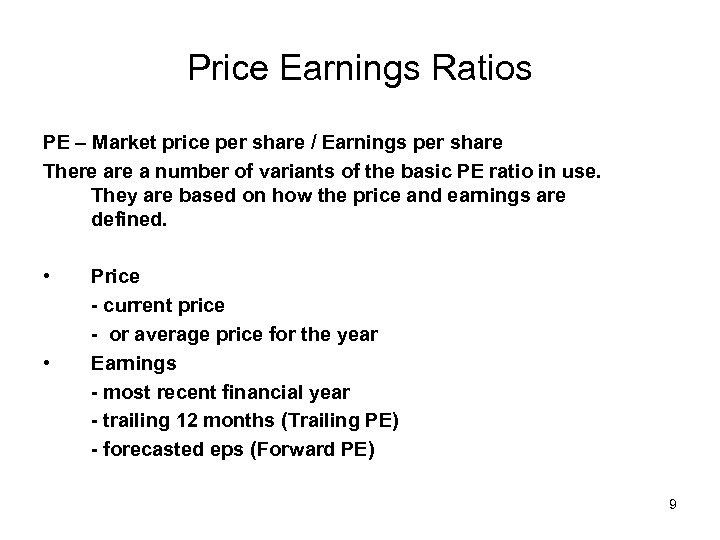Price Earnings Ratios PE – Market price per share / Earnings per share There a number of variants of the basic PE ratio in use. They are based on how the price and earnings are defined. • • Price - current price - or average price for the year Earnings - most recent financial year - trailing 12 months (Trailing PE) - forecasted eps (Forward PE) 9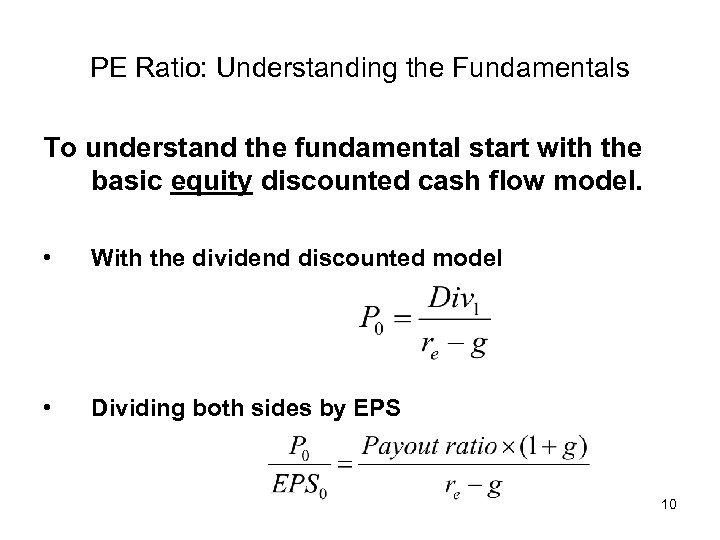PE Ratio: Understanding the Fundamentals To understand the fundamental start with the basic equity discounted cash flow model. • With the dividend discounted model • Dividing both sides by EPS 10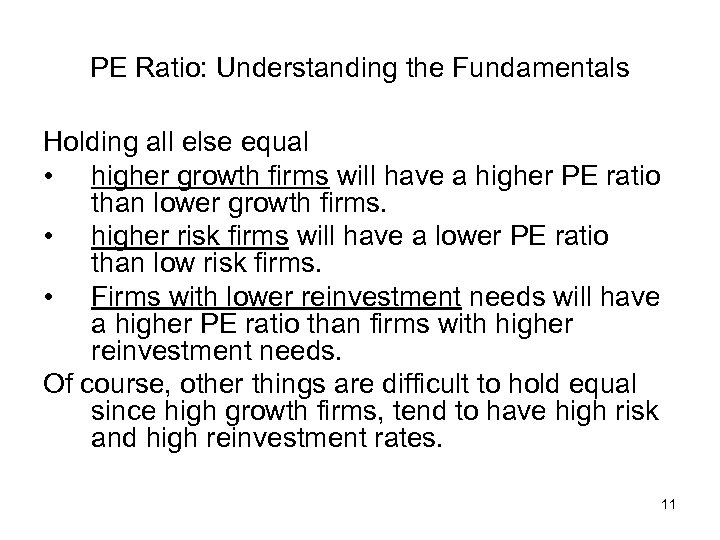PE Ratio: Understanding the Fundamentals Holding all else equal • higher growth firms will have a higher PE ratio than lower growth firms. • higher risk firms will have a lower PE ratio than low risk firms. • Firms with lower reinvestment needs will have a higher PE ratio than firms with higher reinvestment needs. Of course, other things are difficult to hold equal since high growth firms, tend to have high risk and high reinvestment rates. 11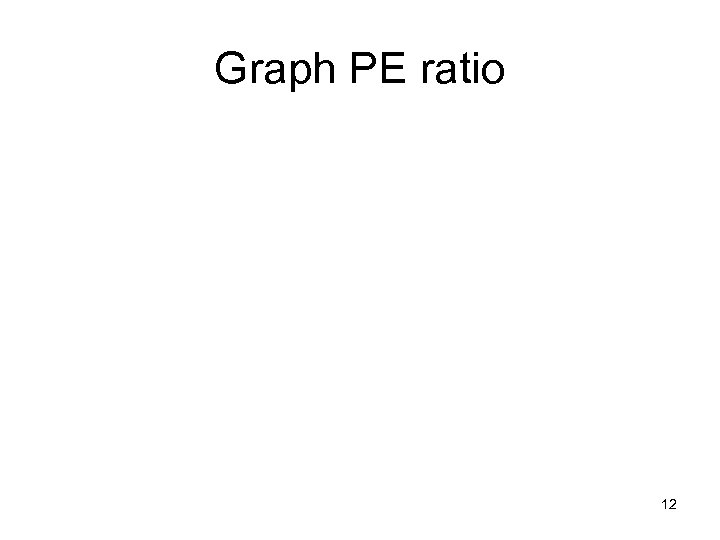Graph PE ratio 12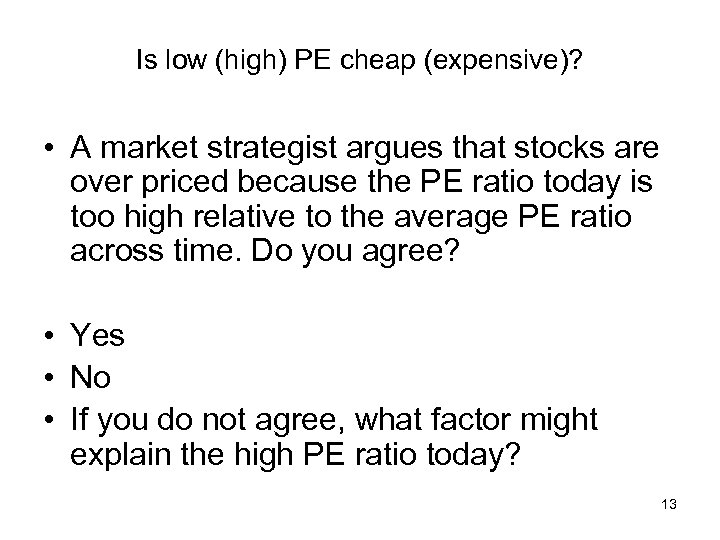Is low (high) PE cheap (expensive)? • A market strategist argues that stocks are over priced because the PE ratio today is too high relative to the average PE ratio across time. Do you agree? • Yes • No • If you do not agree, what factor might explain the high PE ratio today? 13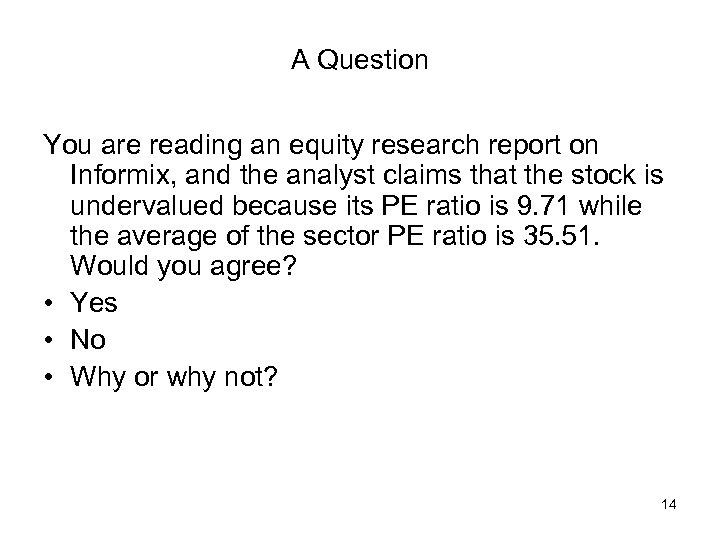A Question You are reading an equity research report on Informix, and the analyst claims that the stock is undervalued because its PE ratio is 9. 71 while the average of the sector PE ratio is 35. 51. Would you agree? • Yes • No • Why or why not? 14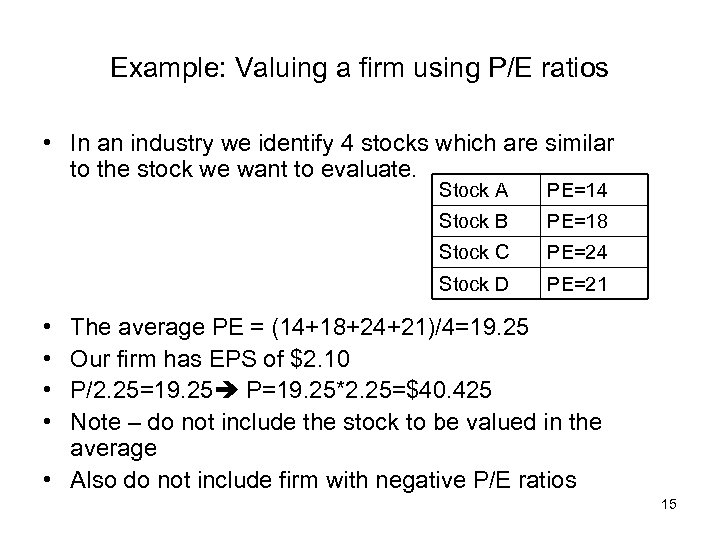Example: Valuing a firm using P/E ratios • In an industry we identify 4 stocks which are similar to the stock we want to evaluate. Stock A PE=14 Stock B PE=18 Stock C PE=24 Stock D PE=21 • • The average PE = (14+18+24+21)/4=19. 25 Our firm has EPS of \$2. 10 P/2. 25=19. 25 P=19. 25*2. 25=\$40. 425 Note – do not include the stock to be valued in the average • Also do not include firm with negative P/E ratios 15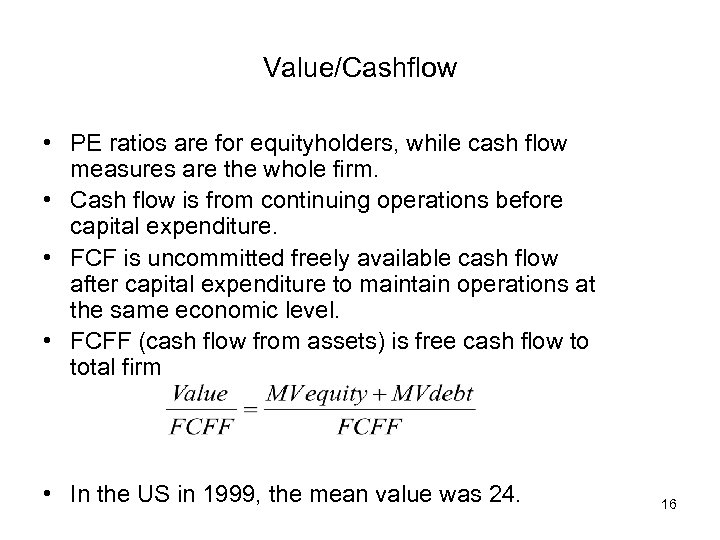Value/Cashflow • PE ratios are for equityholders, while cash flow measures are the whole firm. • Cash flow is from continuing operations before capital expenditure. • FCF is uncommitted freely available cash flow after capital expenditure to maintain operations at the same economic level. • FCFF (cash flow from assets) is free cash flow to total firm • In the US in 1999, the mean value was 24. 16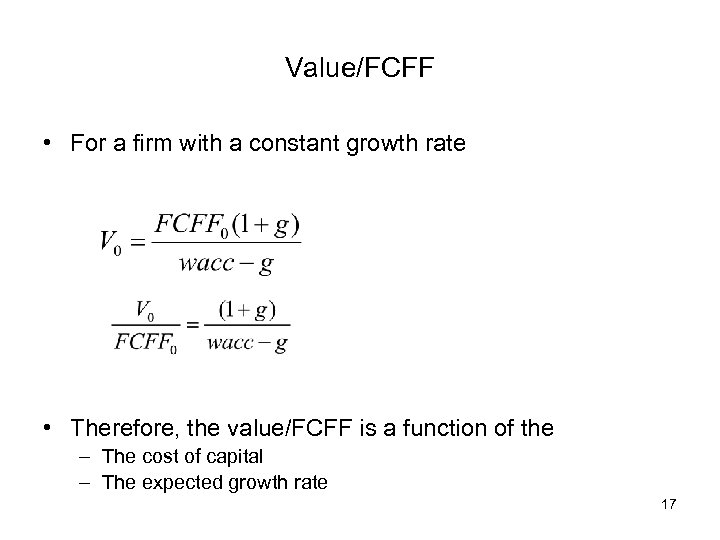Value/FCFF • For a firm with a constant growth rate • Therefore, the value/FCFF is a function of the – The cost of capital – The expected growth rate 17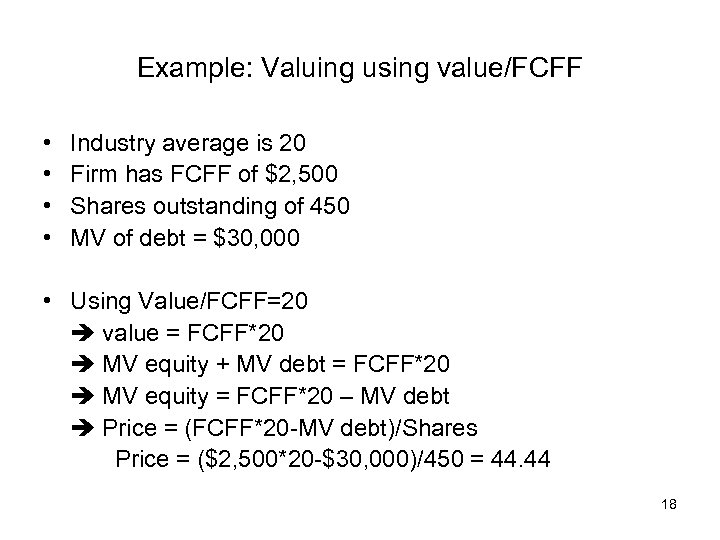Example: Valuing using value/FCFF • • Industry average is 20 Firm has FCFF of \$2, 500 Shares outstanding of 450 MV of debt = \$30, 000 • Using Value/FCFF=20 value = FCFF*20 MV equity + MV debt = FCFF*20 MV equity = FCFF*20 – MV debt Price = (FCFF*20 -MV debt)/Shares Price = (\$2, 500*20 -\$30, 000)/450 = 44. 44 18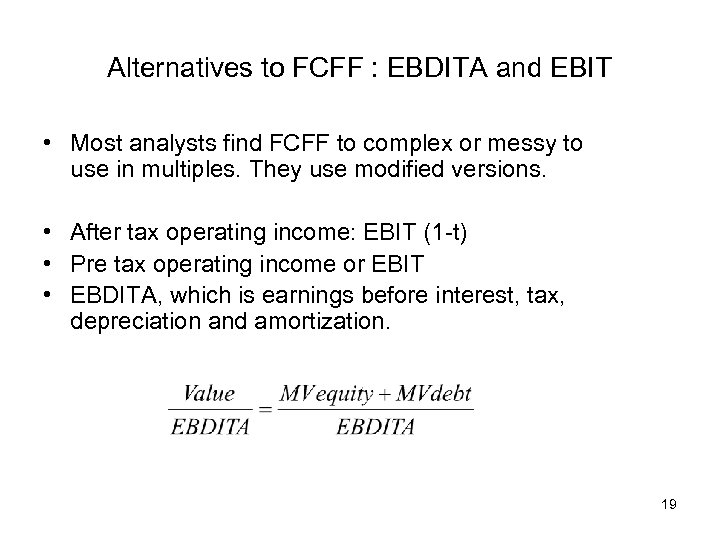Alternatives to FCFF : EBDITA and EBIT • Most analysts find FCFF to complex or messy to use in multiples. They use modified versions. • After tax operating income: EBIT (1 -t) • Pre tax operating income or EBIT • EBDITA, which is earnings before interest, tax, depreciation and amortization. 19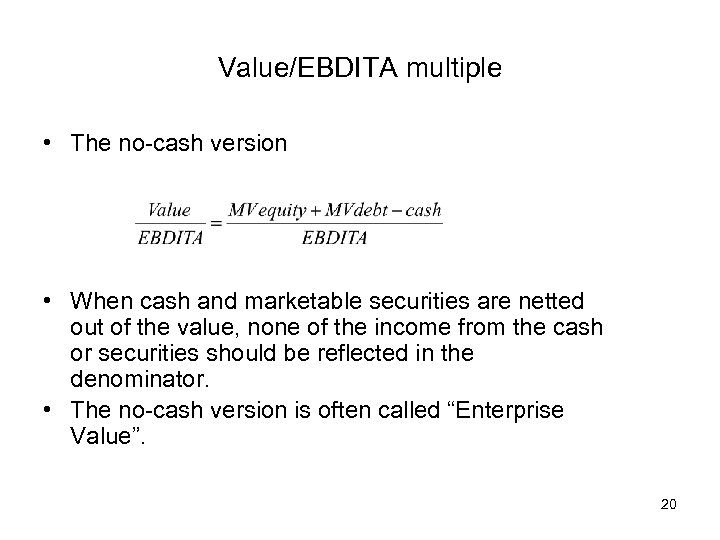Value/EBDITA multiple • The no-cash version • When cash and marketable securities are netted out of the value, none of the income from the cash or securities should be reflected in the denominator. • The no-cash version is often called “Enterprise Value”. 20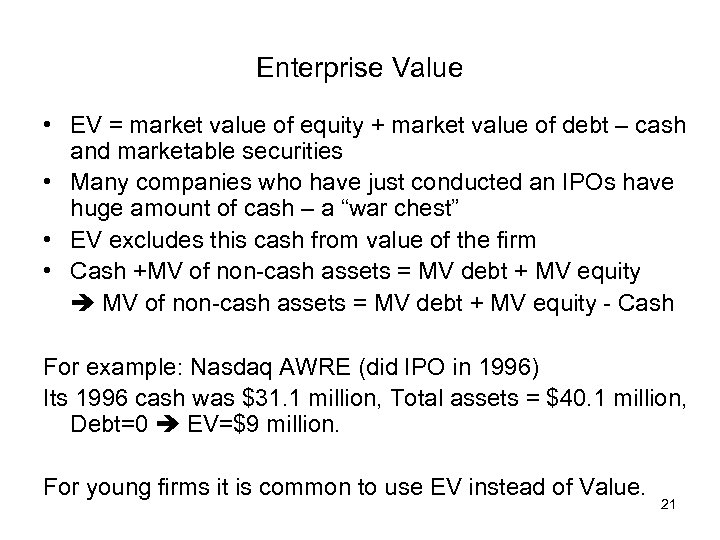Enterprise Value • EV = market value of equity + market value of debt – cash and marketable securities • Many companies who have just conducted an IPOs have huge amount of cash – a “war chest” • EV excludes this cash from value of the firm • Cash +MV of non-cash assets = MV debt + MV equity - Cash For example: Nasdaq AWRE (did IPO in 1996) Its 1996 cash was \$31. 1 million, Total assets = \$40. 1 million, Debt=0 EV=\$9 million. For young firms it is common to use EV instead of Value. 21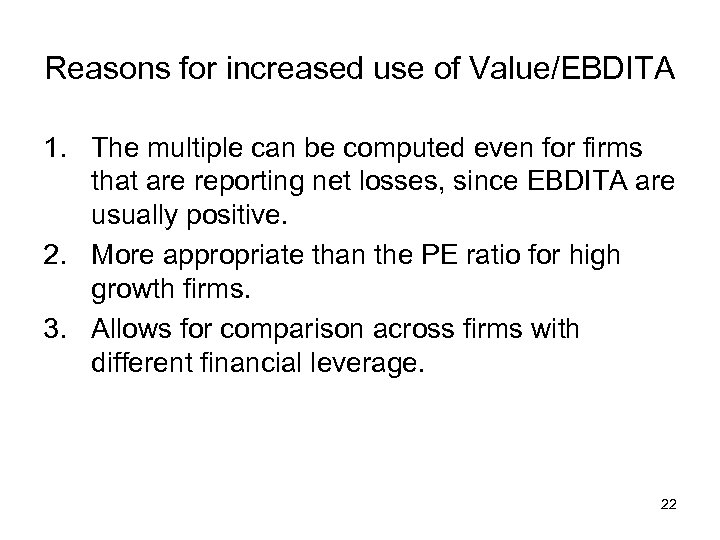Reasons for increased use of Value/EBDITA 1. The multiple can be computed even for firms that are reporting net losses, since EBDITA are usually positive. 2. More appropriate than the PE ratio for high growth firms. 3. Allows for comparison across firms with different financial leverage. 22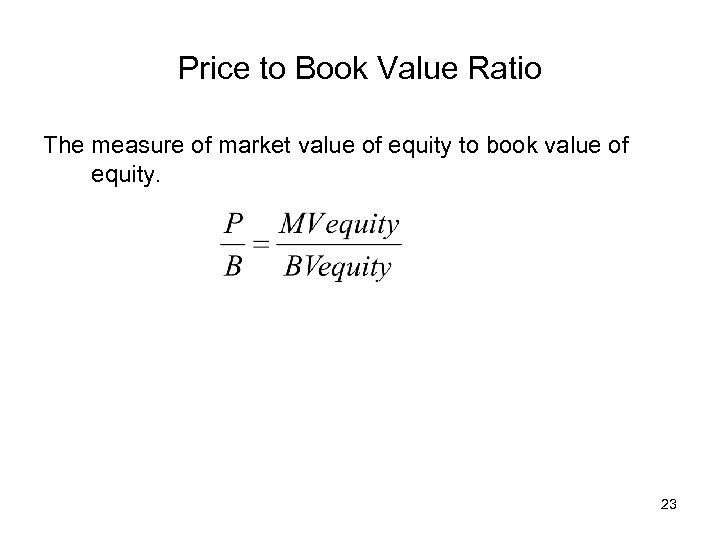Price to Book Value Ratio The measure of market value of equity to book value of equity. 23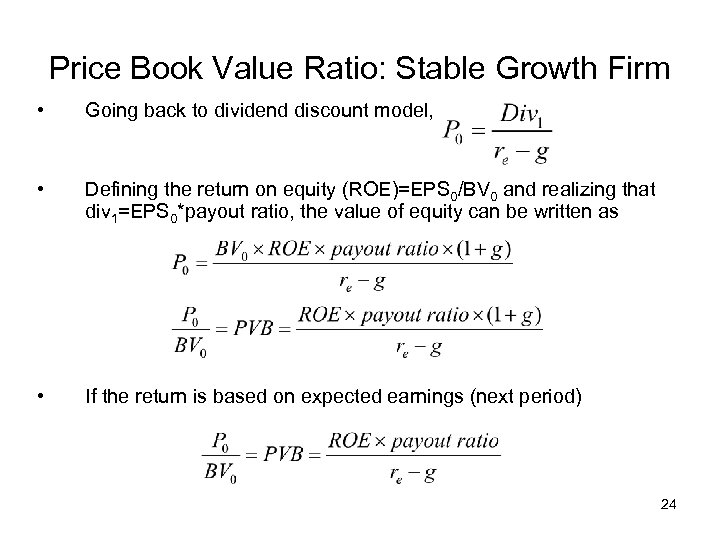Price Book Value Ratio: Stable Growth Firm • Going back to dividend discount model, • Defining the return on equity (ROE)=EPS 0/BV 0 and realizing that div 1=EPS 0*payout ratio, the value of equity can be written as • If the return is based on expected earnings (next period) 24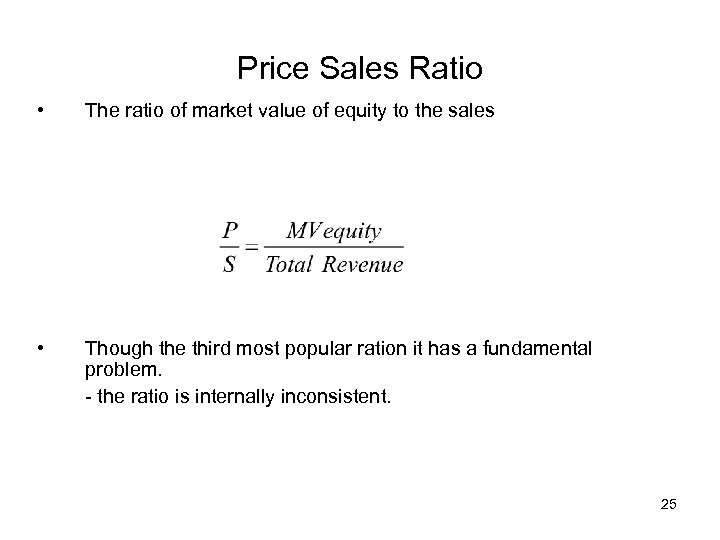Price Sales Ratio • The ratio of market value of equity to the sales • Though the third most popular ration it has a fundamental problem. - the ratio is internally inconsistent. 25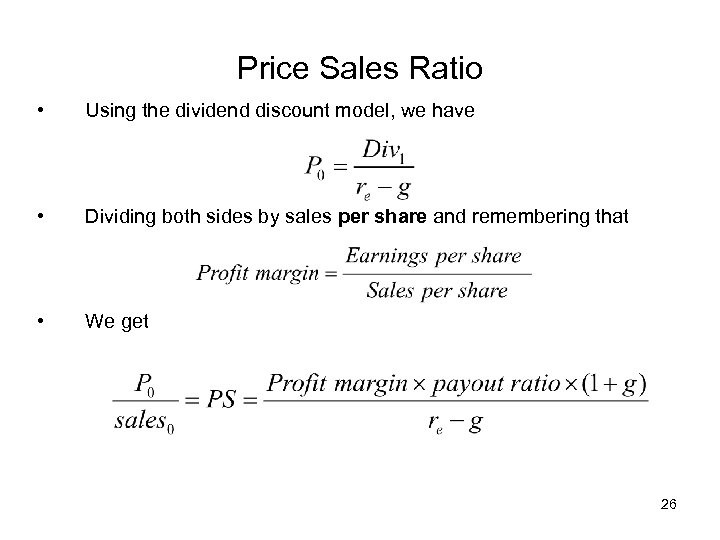Price Sales Ratio • Using the dividend discount model, we have • Dividing both sides by sales per share and remembering that • We get 26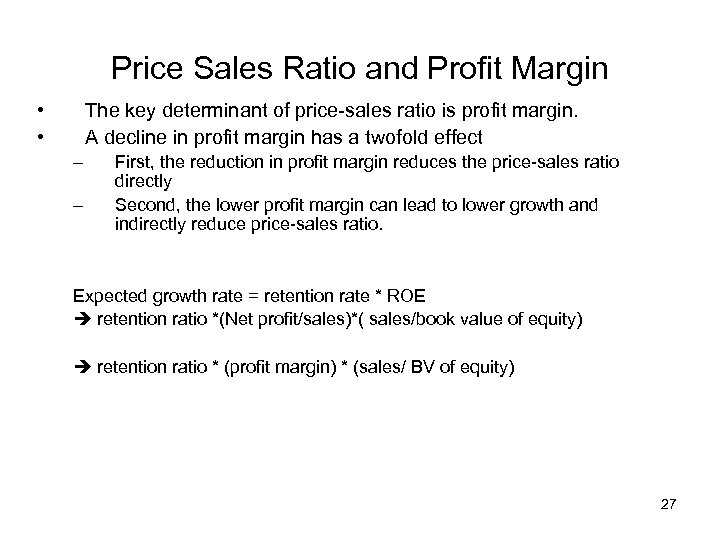Price Sales Ratio and Profit Margin • • The key determinant of price-sales ratio is profit margin. A decline in profit margin has a twofold effect – – First, the reduction in profit margin reduces the price-sales ratio directly Second, the lower profit margin can lead to lower growth and indirectly reduce price-sales ratio. Expected growth rate = retention rate * ROE retention ratio *(Net profit/sales)*( sales/book value of equity) retention ratio * (profit margin) * (sales/ BV of equity) 27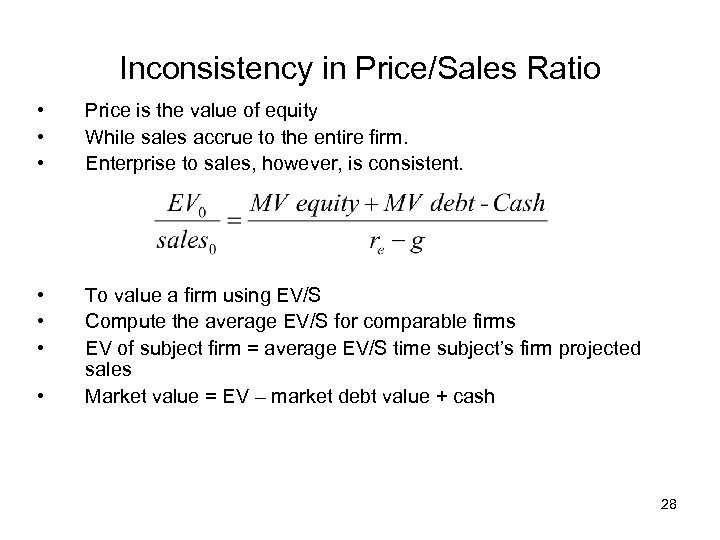Inconsistency in Price/Sales Ratio • • • Price is the value of equity While sales accrue to the entire firm. Enterprise to sales, however, is consistent. • • • To value a firm using EV/S Compute the average EV/S for comparable firms EV of subject firm = average EV/S time subject’s firm projected sales Market value = EV – market debt value + cash • 28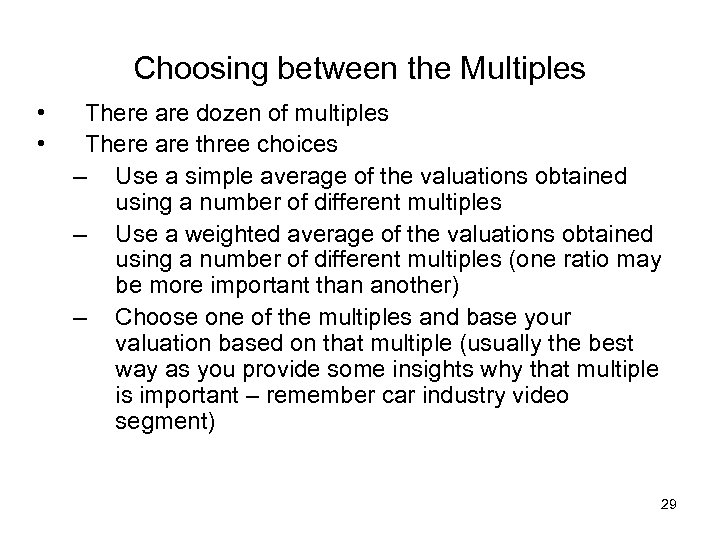Choosing between the Multiples • • There are dozen of multiples There are three choices – Use a simple average of the valuations obtained using a number of different multiples – Use a weighted average of the valuations obtained using a number of different multiples (one ratio may be more important than another) – Choose one of the multiples and base your valuation based on that multiple (usually the best way as you provide some insights why that multiple is important – remember car industry video segment) 29